# Face Generation¶

In this project, you'll use generative adversarial networks to generate new images of faces.

### Get the Data¶

You'll be using two datasets in this project:

• MNIST
• CelebA

Since the celebA dataset is complex and you're doing GANs in a project for the first time, we want you to test your neural network on MNIST before CelebA. Running the GANs on MNIST will allow you to see how well your model trains sooner.

If you're using FloydHub, set data_dir to "/input" and use the FloydHub data ID "R5KrjnANiKVhLWAkpXhNBe".

In :
data_dir = './data'

# FloydHub - Use with data ID "R5KrjnANiKVhLWAkpXhNBe"
#data_dir = '/input'

"""
DON'T MODIFY ANYTHING IN THIS CELL
"""
import helper


Downloading mnist: 9.92MB [00:08, 1.22MB/s]
Extracting mnist: 100%|██████████| 60.0K/60.0K [00:05<00:00, 11.7KFile/s]

Extracting celeba...


## Explore the Data¶

### MNIST¶

As you're aware, the MNIST dataset contains images of handwritten digits. You can view the first number of examples by changing show_n_images.

In :
show_n_images = 25

"""
DON'T MODIFY ANYTHING IN THIS CELL
"""
%matplotlib inline
import os
from glob import glob
from matplotlib import pyplot

mnist_images = helper.get_batch(glob(os.path.join(data_dir, 'mnist/*.jpg'))[:show_n_images], 28, 28, 'L')
pyplot.imshow(helper.images_square_grid(mnist_images, 'L'), cmap='gray')

Out:
<matplotlib.image.AxesImage at 0x7f5180d18240>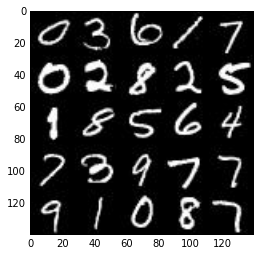### CelebA¶

The CelebFaces Attributes Dataset (CelebA) dataset contains over 200,000 celebrity images with annotations. Since you're going to be generating faces, you won't need the annotations. You can view the first number of examples by changing show_n_images.

In :
show_n_images = 25

"""
DON'T MODIFY ANYTHING IN THIS CELL
"""
mnist_images = helper.get_batch(glob(os.path.join(data_dir, 'img_align_celeba/*.jpg'))[:show_n_images], 28, 28, 'RGB')
pyplot.imshow(helper.images_square_grid(mnist_images, 'RGB'))

Out:
<matplotlib.image.AxesImage at 0x7f5180c9db70>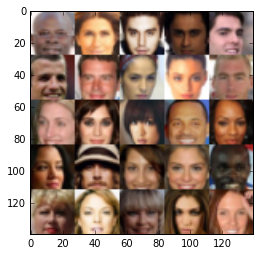## Preprocess the Data¶

Since the project's main focus is on building the GANs, we'll preprocess the data for you. The values of the MNIST and CelebA dataset will be in the range of -0.5 to 0.5 of 28x28 dimensional images. The CelebA images will be cropped to remove parts of the image that don't include a face, then resized down to 28x28.

The MNIST images are black and white images with a single color channel while the CelebA images have 3 color channels (RGB color channel).

## Build the Neural Network¶

You'll build the components necessary to build a GANs by implementing the following functions below:

• model_inputs
• discriminator
• generator
• model_loss
• model_opt
• train

This will check to make sure you have the correct version of TensorFlow and access to a GPU

In :
"""
DON'T MODIFY ANYTHING IN THIS CELL
"""
from distutils.version import LooseVersion
import warnings
import tensorflow as tf

# Check TensorFlow Version
assert LooseVersion(tf.__version__) >= LooseVersion('1.0'), 'Please use TensorFlow version 1.0 or newer.  You are using {}'.format(tf.__version__)
print('TensorFlow Version: {}'.format(tf.__version__))

# Check for a GPU
if not tf.test.gpu_device_name():
warnings.warn('No GPU found. Please use a GPU to train your neural network.')
else:
print('Default GPU Device: {}'.format(tf.test.gpu_device_name()))

TensorFlow Version: 1.0.1
Default GPU Device: /gpu:0


### Input¶

Implement the model_inputs function to create TF Placeholders for the Neural Network. It should create the following placeholders:

• Real input images placeholder with rank 4 using image_width, image_height, and image_channels.
• Z input placeholder with rank 2 using z_dim.
• Learning rate placeholder with rank 0.

Return the placeholders in the following the tuple (tensor of real input images, tensor of z data)

In :
import problem_unittests as tests

def model_inputs(image_width, image_height, image_channels, z_dim):
"""
Create the model inputs
:param image_width: The input image width
:param image_height: The input image height
:param image_channels: The number of image channels
:param z_dim: The dimension of Z
:return: Tuple of (tensor of real input images, tensor of z data, learning rate)
"""
# TODO: Implement Function
inputs_real = tf.placeholder(tf.float32, (None, image_width, image_height, image_channels), name='input_real')
inputs_z = tf.placeholder(tf.float32, (None, z_dim), name='input_z')
learn_rate = tf.placeholder(tf.float32, name='learn_rate')
return inputs_real, inputs_z, learn_rate

"""
DON'T MODIFY ANYTHING IN THIS CELL THAT IS BELOW THIS LINE
"""
tests.test_model_inputs(model_inputs)

Tests Passed


### Discriminator¶

Implement discriminator to create a discriminator neural network that discriminates on images. This function should be able to reuse the variabes in the neural network. Use tf.variable_scope with a scope name of "discriminator" to allow the variables to be reused. The function should return a tuple of (tensor output of the generator, tensor logits of the generator).

In :
def discriminator(images, reuse=False):
"""
Create the discriminator network
:param image: Tensor of input image(s)
:param reuse: Boolean if the weights should be reused
:return: Tuple of (tensor output of the discriminator, tensor logits of the discriminator)
"""
# TODO: Implement Function
alpha = 0.2
with tf.variable_scope('discriminator', reuse=reuse):
# Input layer is 28x28x3
x1 = tf.layers.conv2d(images, 64, 5, strides=2, padding='same')
relu1 = tf.maximum(alpha * x1, x1)
# 14x14x32

x2 = tf.layers.conv2d(relu1, 128, 5, strides=2, padding='same')
bn2 = tf.layers.batch_normalization(x2, training=True)
relu2 = tf.maximum(alpha * bn2, bn2)
# 7x7x128

x3 = tf.layers.conv2d(relu2, 256, 5, strides=2, padding='same')
bn3 = tf.layers.batch_normalization(x3, training=True)
relu3 = tf.maximum(alpha * bn3, bn3)
# 4x4x256

# Flatten it
flat = tf.reshape(relu3, (-1, 4*4*256))
logits = tf.layers.dense(flat, 1)
out = tf.sigmoid(logits)

return out, logits

"""
DON'T MODIFY ANYTHING IN THIS CELL THAT IS BELOW THIS LINE
"""
tests.test_discriminator(discriminator, tf)

Tests Passed


### Generator¶

Implement generator to generate an image using z. This function should be able to reuse the variabes in the neural network. Use tf.variable_scope with a scope name of "generator" to allow the variables to be reused. The function should return the generated 28 x 28 x out_channel_dim images.

In :
def generator(z, out_channel_dim, is_train=True):
"""
Create the generator network
:param z: Input z
:param out_channel_dim: The number of channels in the output image
:param is_train: Boolean if generator is being used for training
:return: The tensor output of the generator
"""
# TODO: Implement Function
alpha = 0.2
with tf.variable_scope('generator', reuse=not is_train):
# First fully connected layer
x1 = tf.layers.dense(z, 2*2*512)
# Reshape it to start the convolutional stack
x1 = tf.reshape(x1, (-1, 2, 2, 512))
# x1 = tf.layers.batch_normalization(x1, training=is_train)
x1 = tf.maximum(alpha * x1, x1)
# 4x4x512 now

x2 = tf.layers.conv2d_transpose(x1, 256, 5, strides=2, padding='valid')
x2 = tf.layers.batch_normalization(x2, training=is_train)
x2 = tf.maximum(alpha * x2, x2)
# 16x16x256 now
x3 = tf.layers.conv2d_transpose(x2, 128, 5, strides=2, padding='same')
x3 = tf.layers.batch_normalization(x3, training=is_train)
x3 = tf.maximum(alpha * x3, x3)

# Output layer
logits = tf.layers.conv2d_transpose(x3, out_channel_dim, 5, strides=2, padding='same')
# 32x32x3 now

#logits = tf.slice(logits, [0, 2, 2, 0], [-1, 28, 28, -1])
out = tf.tanh(logits)

return out

"""
DON'T MODIFY ANYTHING IN THIS CELL THAT IS BELOW THIS LINE
"""
tests.test_generator(generator, tf)

Tests Passed


### Loss¶

Implement model_loss to build the GANs for training and calculate the loss. The function should return a tuple of (discriminator loss, generator loss). Use the following functions you implemented:

• discriminator(images, reuse=False)
• generator(z, out_channel_dim, is_train=True)
In :
def model_loss(input_real, input_z, out_channel_dim):
"""
Get the loss for the discriminator and generator
:param input_real: Images from the real dataset
:param input_z: Z input
:param out_channel_dim: The number of channels in the output image
:return: A tuple of (discriminator loss, generator loss)
"""
# TODO: Implement Function
g_model = generator(input_z, out_channel_dim, is_train=True)
d_model_real, d_logits_real = discriminator(input_real, reuse=False)
d_model_fake, d_logits_fake = discriminator(g_model, reuse=True)

d_loss_real = tf.reduce_mean(
tf.nn.sigmoid_cross_entropy_with_logits(logits=d_logits_real, labels=tf.ones_like(d_model_real)))
d_loss_fake = tf.reduce_mean(
tf.nn.sigmoid_cross_entropy_with_logits(logits=d_logits_fake, labels=tf.zeros_like(d_model_fake)))
g_loss = tf.reduce_mean(
tf.nn.sigmoid_cross_entropy_with_logits(logits=d_logits_fake, labels=tf.ones_like(d_model_fake)))

d_loss = d_loss_real + d_loss_fake

return d_loss, g_loss

"""
DON'T MODIFY ANYTHING IN THIS CELL THAT IS BELOW THIS LINE
"""
tests.test_model_loss(model_loss)

Tests Passed


### Optimization¶

Implement model_opt to create the optimization operations for the GANs. Use tf.trainable_variables to get all the trainable variables. Filter the variables with names that are in the discriminator and generator scope names. The function should return a tuple of (discriminator training operation, generator training operation).

In :
def model_opt(d_loss, g_loss, learning_rate, beta1):
"""
Get optimization operations
:param d_loss: Discriminator loss Tensor
:param g_loss: Generator loss Tensor
:param learning_rate: Learning Rate Placeholder
:param beta1: The exponential decay rate for the 1st moment in the optimizer
:return: A tuple of (discriminator training operation, generator training operation)
"""
# TODO: Implement Function
# Get weights and bias to update
t_vars = tf.trainable_variables()
d_vars = [var for var in t_vars if var.name.startswith('discriminator')]
g_vars = [var for var in t_vars if var.name.startswith('generator')]

# Optimize

with tf.control_dependencies(tf.get_collection(tf.GraphKeys.UPDATE_OPS)):

return d_train_opt, g_train_opt

"""
DON'T MODIFY ANYTHING IN THIS CELL THAT IS BELOW THIS LINE
"""
tests.test_model_opt(model_opt, tf)

Tests Passed


## Neural Network Training¶

### Show Output¶

Use this function to show the current output of the generator during training. It will help you determine how well the GANs is training.

In :
"""
DON'T MODIFY ANYTHING IN THIS CELL
"""
import numpy as np

def show_generator_output(sess, n_images, input_z, out_channel_dim, image_mode):
"""
Show example output for the generator
:param sess: TensorFlow session
:param n_images: Number of Images to display
:param input_z: Input Z Tensor
:param out_channel_dim: The number of channels in the output image
:param image_mode: The mode to use for images ("RGB" or "L")
"""
cmap = None if image_mode == 'RGB' else 'gray'
z_dim = input_z.get_shape().as_list()[-1]
example_z = np.random.uniform(-1, 1, size=[n_images, z_dim])

samples = sess.run(
generator(input_z, out_channel_dim, False),
feed_dict={input_z: example_z})

images_grid = helper.images_square_grid(samples, image_mode)
pyplot.imshow(images_grid, cmap=cmap)
pyplot.show()


### Train¶

Implement train to build and train the GANs. Use the following functions you implemented:

• model_inputs(image_width, image_height, image_channels, z_dim)
• model_loss(input_real, input_z, out_channel_dim)
• model_opt(d_loss, g_loss, learning_rate, beta1)

Use the show_generator_output to show generator output while you train. Running show_generator_output for every batch will drastically increase training time and increase the size of the notebook. It's recommended to print the generator output every 100 batches.

In :
def train(epoch_count, batch_size, z_dim, learning_rate, beta1, get_batches, data_shape, data_image_mode):
"""
Train the GAN
:param epoch_count: Number of epochs
:param batch_size: Batch Size
:param z_dim: Z dimension
:param learning_rate: Learning Rate
:param beta1: The exponential decay rate for the 1st moment in the optimizer
:param get_batches: Function to get batches
:param data_shape: Shape of the data
:param data_image_mode: The image mode to use for images ("RGB" or "L")
"""
# TODO: Build Model
n_samples, width, height, channels = data_shape
input_real, input_z, learn_rate = model_inputs(width, height, channels, z_dim)
d_loss, g_loss = model_loss(input_real, input_z, channels)
d_opt, g_opt = model_opt(d_loss, g_loss, learn_rate, beta1)

steps = 0
show_every = 50
print_every = 10
with tf.Session() as sess:
sess.run(tf.global_variables_initializer())
for epoch_i in range(epoch_count):
for batch_images in get_batches(batch_size):
batch_images *= 2
# TODO: Train Model
steps += 1
# Sample random noise for G
batch_z = np.random.uniform(-1, 1, size=(batch_size, z_dim))

# Run optimizers
_ = sess.run(d_opt, feed_dict={input_real: batch_images, input_z: batch_z, learn_rate: learning_rate})
_ = sess.run(g_opt, feed_dict={input_real: batch_images, input_z: batch_z, learn_rate: learning_rate})

if steps % show_every == 0:
n_images = 16
show_generator_output(sess, n_images, input_z, channels, data_image_mode)

if steps % print_every == 0:
train_loss_d = d_loss.eval({input_z: batch_z, input_real: batch_images})
train_loss_g = g_loss.eval({input_z: batch_z})

print("Epoch {}/{}...".format(epoch_i, epoch_count),
"Discriminator Loss: {:.4f}...".format(train_loss_d),
"Generator Loss: {:.4f}".format(train_loss_g))



### MNIST¶

Test your GANs architecture on MNIST. After 2 epochs, the GANs should be able to generate images that look like handwritten digits. Make sure the loss of the generator is lower than the loss of the discriminator or close to 0.

In :
batch_size = 64
z_dim = 100
learning_rate = 0.0004
beta1 = 0.5

"""
DON'T MODIFY ANYTHING IN THIS CELL THAT IS BELOW THIS LINE
"""
epochs = 2

mnist_dataset = helper.Dataset('mnist', glob(os.path.join(data_dir, 'mnist/*.jpg')))
with tf.Graph().as_default():
train(epochs, batch_size, z_dim, learning_rate, beta1, mnist_dataset.get_batches,
mnist_dataset.shape, mnist_dataset.image_mode)

Epoch 0/2... Discriminator Loss: 0.0608... Generator Loss: 3.1503
Epoch 0/2... Discriminator Loss: 0.0979... Generator Loss: 3.4716
Epoch 0/2... Discriminator Loss: 0.0030... Generator Loss: 9.2256
Epoch 0/2... Discriminator Loss: 5.7647... Generator Loss: 0.0048
Epoch 0/2... Discriminator Loss: 0.2171... Generator Loss: 3.2467
Epoch 0/2... Discriminator Loss: 0.8013... Generator Loss: 3.7034
Epoch 0/2... Discriminator Loss: 0.3399... Generator Loss: 2.7230
Epoch 0/2... Discriminator Loss: 0.0268... Generator Loss: 6.7176
Epoch 0/2... Discriminator Loss: 0.8812... Generator Loss: 2.5627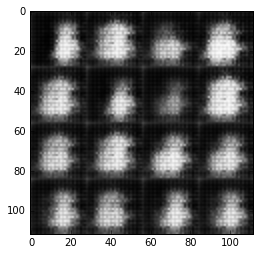Epoch 0/2... Discriminator Loss: 0.3031... Generator Loss: 3.4149
Epoch 0/2... Discriminator Loss: 0.9019... Generator Loss: 0.6205
Epoch 0/2... Discriminator Loss: 0.3335... Generator Loss: 1.8543
Epoch 0/2... Discriminator Loss: 0.4491... Generator Loss: 1.7703
Epoch 0/2... Discriminator Loss: 0.1433... Generator Loss: 3.0178
Epoch 0/2... Discriminator Loss: 0.0842... Generator Loss: 3.6386
Epoch 0/2... Discriminator Loss: 0.3066... Generator Loss: 2.7727
Epoch 0/2... Discriminator Loss: 0.1537... Generator Loss: 4.8310
Epoch 0/2... Discriminator Loss: 0.3486... Generator Loss: 1.7167
Epoch 0/2... Discriminator Loss: 0.0343... Generator Loss: 4.6618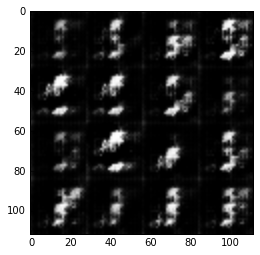Epoch 0/2... Discriminator Loss: 1.0076... Generator Loss: 9.1079
Epoch 0/2... Discriminator Loss: 0.2767... Generator Loss: 4.4248
Epoch 0/2... Discriminator Loss: 0.1400... Generator Loss: 3.3659
Epoch 0/2... Discriminator Loss: 0.1269... Generator Loss: 3.2889
Epoch 0/2... Discriminator Loss: 0.0839... Generator Loss: 5.6814
Epoch 0/2... Discriminator Loss: 0.2459... Generator Loss: 2.9233
Epoch 0/2... Discriminator Loss: 0.2866... Generator Loss: 4.9421
Epoch 0/2... Discriminator Loss: 0.0688... Generator Loss: 3.9421
Epoch 0/2... Discriminator Loss: 0.0692... Generator Loss: 6.6158
Epoch 0/2... Discriminator Loss: 0.1371... Generator Loss: 3.8557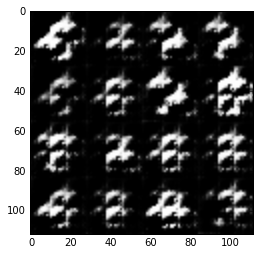Epoch 0/2... Discriminator Loss: 0.2902... Generator Loss: 9.3342
Epoch 0/2... Discriminator Loss: 0.1365... Generator Loss: 3.0919
Epoch 0/2... Discriminator Loss: 0.2543... Generator Loss: 3.7096
Epoch 0/2... Discriminator Loss: 0.2446... Generator Loss: 3.0415
Epoch 0/2... Discriminator Loss: 0.1877... Generator Loss: 2.4318
Epoch 0/2... Discriminator Loss: 0.1828... Generator Loss: 2.8904
Epoch 0/2... Discriminator Loss: 2.7937... Generator Loss: 11.0747
Epoch 0/2... Discriminator Loss: 0.1638... Generator Loss: 2.6556
Epoch 0/2... Discriminator Loss: 0.1588... Generator Loss: 3.0368
Epoch 0/2... Discriminator Loss: 0.9883... Generator Loss: 0.7067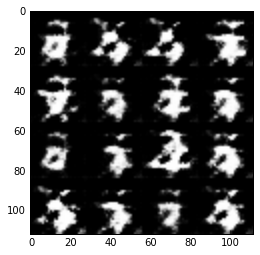Epoch 0/2... Discriminator Loss: 0.3295... Generator Loss: 2.4852
Epoch 0/2... Discriminator Loss: 0.2234... Generator Loss: 2.7936
Epoch 0/2... Discriminator Loss: 0.1038... Generator Loss: 3.6885
Epoch 0/2... Discriminator Loss: 0.6614... Generator Loss: 1.0463
Epoch 0/2... Discriminator Loss: 0.2077... Generator Loss: 3.0942
Epoch 0/2... Discriminator Loss: 0.4120... Generator Loss: 1.5384
Epoch 0/2... Discriminator Loss: 0.2242... Generator Loss: 2.7151
Epoch 0/2... Discriminator Loss: 0.1506... Generator Loss: 3.3552
Epoch 0/2... Discriminator Loss: 0.2380... Generator Loss: 2.9878
Epoch 0/2... Discriminator Loss: 0.1079... Generator Loss: 3.4102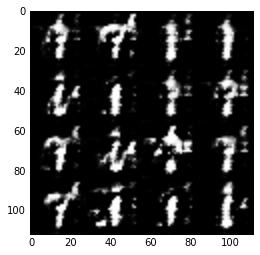Epoch 0/2... Discriminator Loss: 0.3529... Generator Loss: 1.5792
Epoch 0/2... Discriminator Loss: 0.2684... Generator Loss: 3.4172
Epoch 0/2... Discriminator Loss: 0.2112... Generator Loss: 4.3204
Epoch 0/2... Discriminator Loss: 0.4421... Generator Loss: 2.3349
Epoch 0/2... Discriminator Loss: 0.3436... Generator Loss: 2.1159
Epoch 0/2... Discriminator Loss: 0.1572... Generator Loss: 3.3572
Epoch 0/2... Discriminator Loss: 0.3785... Generator Loss: 3.6073
Epoch 0/2... Discriminator Loss: 0.2154... Generator Loss: 4.2024
Epoch 0/2... Discriminator Loss: 0.2467... Generator Loss: 5.7378
Epoch 0/2... Discriminator Loss: 0.6071... Generator Loss: 1.7398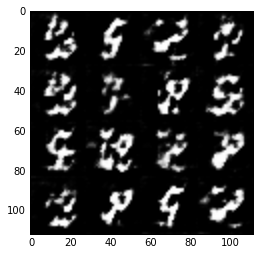Epoch 0/2... Discriminator Loss: 0.1449... Generator Loss: 2.6788
Epoch 0/2... Discriminator Loss: 0.1759... Generator Loss: 3.2335
Epoch 0/2... Discriminator Loss: 0.4619... Generator Loss: 1.4733
Epoch 0/2... Discriminator Loss: 0.8361... Generator Loss: 5.8598
Epoch 0/2... Discriminator Loss: 0.1973... Generator Loss: 3.2512
Epoch 0/2... Discriminator Loss: 0.4016... Generator Loss: 2.3892
Epoch 0/2... Discriminator Loss: 0.2318... Generator Loss: 2.7721
Epoch 0/2... Discriminator Loss: 0.3038... Generator Loss: 2.6730
Epoch 0/2... Discriminator Loss: 0.5847... Generator Loss: 1.4260
Epoch 0/2... Discriminator Loss: 0.4247... Generator Loss: 1.7036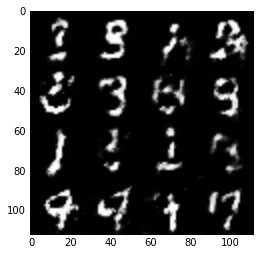Epoch 0/2... Discriminator Loss: 0.5470... Generator Loss: 1.5805
Epoch 0/2... Discriminator Loss: 0.5785... Generator Loss: 2.9843
Epoch 0/2... Discriminator Loss: 0.5359... Generator Loss: 3.5175
Epoch 0/2... Discriminator Loss: 0.2551... Generator Loss: 2.4220
Epoch 0/2... Discriminator Loss: 0.2674... Generator Loss: 2.4648
Epoch 0/2... Discriminator Loss: 0.6154... Generator Loss: 1.5400
Epoch 0/2... Discriminator Loss: 0.4237... Generator Loss: 1.7063
Epoch 0/2... Discriminator Loss: 0.5761... Generator Loss: 1.3921
Epoch 0/2... Discriminator Loss: 0.6138... Generator Loss: 1.2664
Epoch 0/2... Discriminator Loss: 0.6664... Generator Loss: 2.1740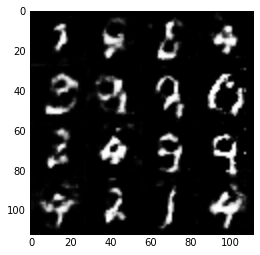Epoch 0/2... Discriminator Loss: 0.5312... Generator Loss: 1.7956
Epoch 0/2... Discriminator Loss: 1.7098... Generator Loss: 0.4344
Epoch 0/2... Discriminator Loss: 0.7453... Generator Loss: 1.4479
Epoch 0/2... Discriminator Loss: 0.6457... Generator Loss: 1.5881
Epoch 0/2... Discriminator Loss: 0.5869... Generator Loss: 1.5640
Epoch 0/2... Discriminator Loss: 0.9253... Generator Loss: 0.9944
Epoch 0/2... Discriminator Loss: 0.8732... Generator Loss: 0.8791
Epoch 0/2... Discriminator Loss: 0.8276... Generator Loss: 1.2919
Epoch 0/2... Discriminator Loss: 0.5979... Generator Loss: 1.2404
Epoch 0/2... Discriminator Loss: 0.4910... Generator Loss: 1.8017